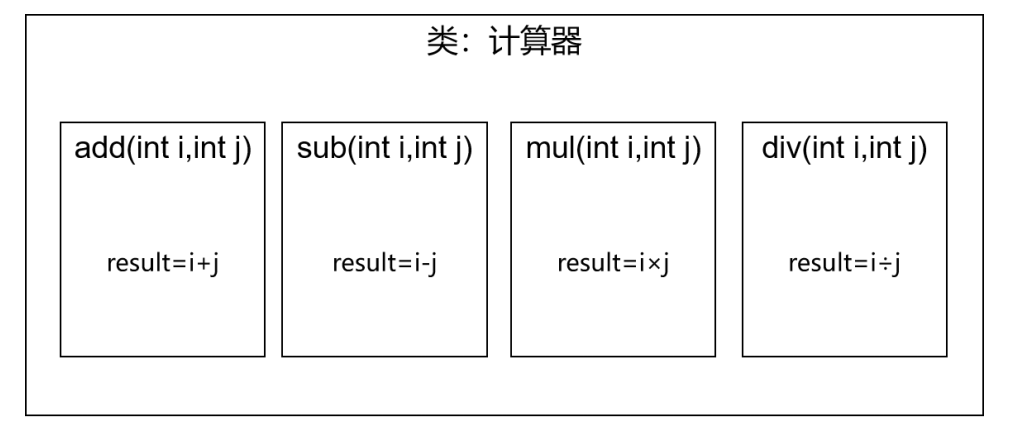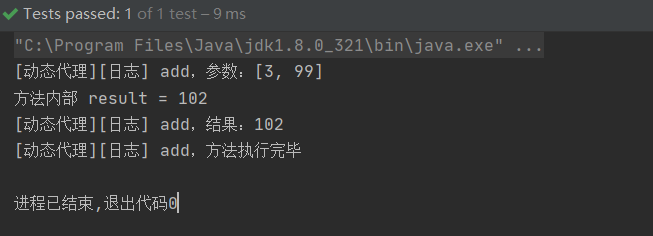﻿ Spring设计模式中代理模式详细讲解_java_脚本之家
java# Spring设计模式中代理模式详细讲解

## 一、场景模拟

①声明接口

```public interface Calculator {
int sub(int i, int j);
int mul(int i, int j);
int div(int i, int j);
}```

②创建实现类```public class CalculatorPureImpl implements Calculator {
@Override
public int add(int i, int j) {
int result = i + j;
System.out.println("方法内部 result = " + result);
return result;
}
@Override
public int sub(int i, int j) {
int result = i - j;
System.out.println("方法内部 result = " + result);
return result;
}
@Override
public int mul(int i, int j) {
int result = i * j;
System.out.println("方法内部 result = " + result);
return result;
}
@Override
public int div(int i, int j) {
int result = i / j;
System.out.println("方法内部 result = " + result);
return result;
}
}```

③创建带日志功能的实现类```public class CalculatorPureImpl implements Calculator {
@Override
public int add(int i, int j) {
System.out.println("[日志] add 方法开始了，参数是：" + i + "," + j);
int result = i + j;
System.out.println("方法内部 result = " + result);
return result;
}
@Override
public int sub(int i, int j) {
System.out.println("[日志] sub 方法开始了，参数是：" + i + "," + j);
int result = i - j;
System.out.println("方法内部 result = " + result);
System.out.println("[日志] sub 方法结束了，结果是：" + result);
return result;
}
@Override
public int mul(int i, int j) {
System.out.println("[日志] mul 方法开始了，参数是：" + i + "," + j);
int result = i * j;
System.out.println("方法内部 result = " + result);
System.out.println("[日志] mul 方法结束了，结果是：" + result);
return result;
}
@Override
public int div(int i, int j) {
System.out.println("[日志] div 方法开始了，参数是：" + i + "," + j);
int result = i / j;
System.out.println("方法内部 result = " + result);
System.out.println("[日志] div 方法结束了，结果是：" + result);
return result;
}
}```

## 二、提出问题

①现有代码缺陷

• 对核心业务功能有干扰，导致程序员在开发核心业务功能时分散了精力
• 附加功能分散在各个业务功能方法中，不利于统一维护

②解决思路

③困难

## 三、代理模式

①介绍 二十三种设计模式中的一种，属于结构型模式。它的作用就是通过提供一个代理类，让我们在调用目标方法的时候，不再是直接对目标方法进行调用，而是通过代理类间接 调用。让不属于目标方法核心逻辑 的代码从目标方法中剥离出来—— 解耦 。调用目标方法时先调用代理对象的方法，减少对目标方法的调用和打扰，同时让附加功能能够集中在一起也有利于统一维护。②生活中的代理

• 广告商找大明星拍广告需要经过经纪人
• 合作伙伴找大老板谈合作要约见面时间需要经过秘书
• 房产中介是买卖双方的代理

③相关术语

• 代理：将非核心逻辑剥离出来以后，封装这些非核心逻辑的类、对象、方法。
• 目标：被代理“套用”了非核心逻辑代码的类、对象、方法。

### 1.静态代理

```public class CalculatorStaticProxy implements Calculator {
// 将被代理的目标对象声明为成员变量
private Calculator target;
public CalculatorStaticProxy(Calculator target) {
this.target = target;
}
@Override
public int add(int i, int j) {
System.out.println("[日志] add 方法开始了，参数是：" + i + "," + j);
return result;
}
@Override
public int sub(int i, int j) {
System.out.println("[日志] sub 方法开始了，参数是：" + i + "," + j);
int result = target.sub(i,j);
System.out.println("[日志] sub 方法结束了，结果是：" + result);
return result;
}
@Override
public int mul(int i, int j) {
System.out.println("[日志] mul 方法开始了，参数是：" + i + "," + j);
int result = target.mul(i,j);
System.out.println("[日志] mul 方法结束了，结果是：" + result);
return result;
}
@Override
public int div(int i, int j) {
System.out.println("[日志] div 方法开始了，参数是：" + i + "," + j);
int result = target.div(i,j);
System.out.println("[日志] div 方法结束了，结果是：" + result);
return result;
}
}```

```public class CalculatorPureImpl implements Calculator {
@Override
public int add(int i, int j) {
int result = i + j;
System.out.println("方法内部 result = " + result);
return result;
}
@Override
public int sub(int i, int j) {
int result = i - j;
System.out.println("方法内部 result = " + result);
return result;
}
@Override
public int mul(int i, int j) {
int result = i * j;
System.out.println("方法内部 result = " + result);
return result;
}
@Override
public int div(int i, int j) {
int result = i / j;
System.out.println("方法内部 result = " + result);
return result;
}
}```

```    public void test(){
CalculatorStaticProxy proxy = new CalculatorStaticProxy(new CalculatorPureImpl());
}```### 2.动态代理```public class ProxyFactory {
Object target;
public ProxyFactory(Object target) {
this.target = target;
}
public Object getProxy(){
/**
* newProxyInstance()：创建一个代理实例
* 其中有三个参数：
* 2、interfaces：目标对象实现的所有接口的class对象所组成的数组
* 3、invocationHandler：设置代理对象实现目标对象方法的过程，即代理类中如何重写接口中的抽象方法
*/
Class<?>[] interfaces = target.getClass().getInterfaces();
InvocationHandler invocationHandler = new InvocationHandler() {
@Override
public Object invoke(Object proxy, Method method, Object[] args) throws Throwable {
/**
* proxy：代理对象
* method：代理对象需要实现的方法，即其中需要重写的方法
* args：method所对应方法的参数
*/
Object result = null;
try {
System.out.println("[动态代理][日志] "+method.getName()+"，参数："+ Arrays.toString(args));
result= method.invoke(target, args);
System.out.println("[动态代理][日志] "+method.getName()+"，结果："+ result);
}catch (Exception e){
e.printStackTrace();
System.out.println("[动态代理][日志] "+method.getName()+"，异常："+e.getMessage());
}finally {
System.out.println("[动态代理][日志] "+method.getName()+"，方法执行完毕");
}
return result;
}
};
}
}```

```    public void test(){
ProxyFactory proxyFactory = new ProxyFactory(new CalculatorPureImpl());
Calculator proxy = (Calculator)proxyFactory.getProxy();